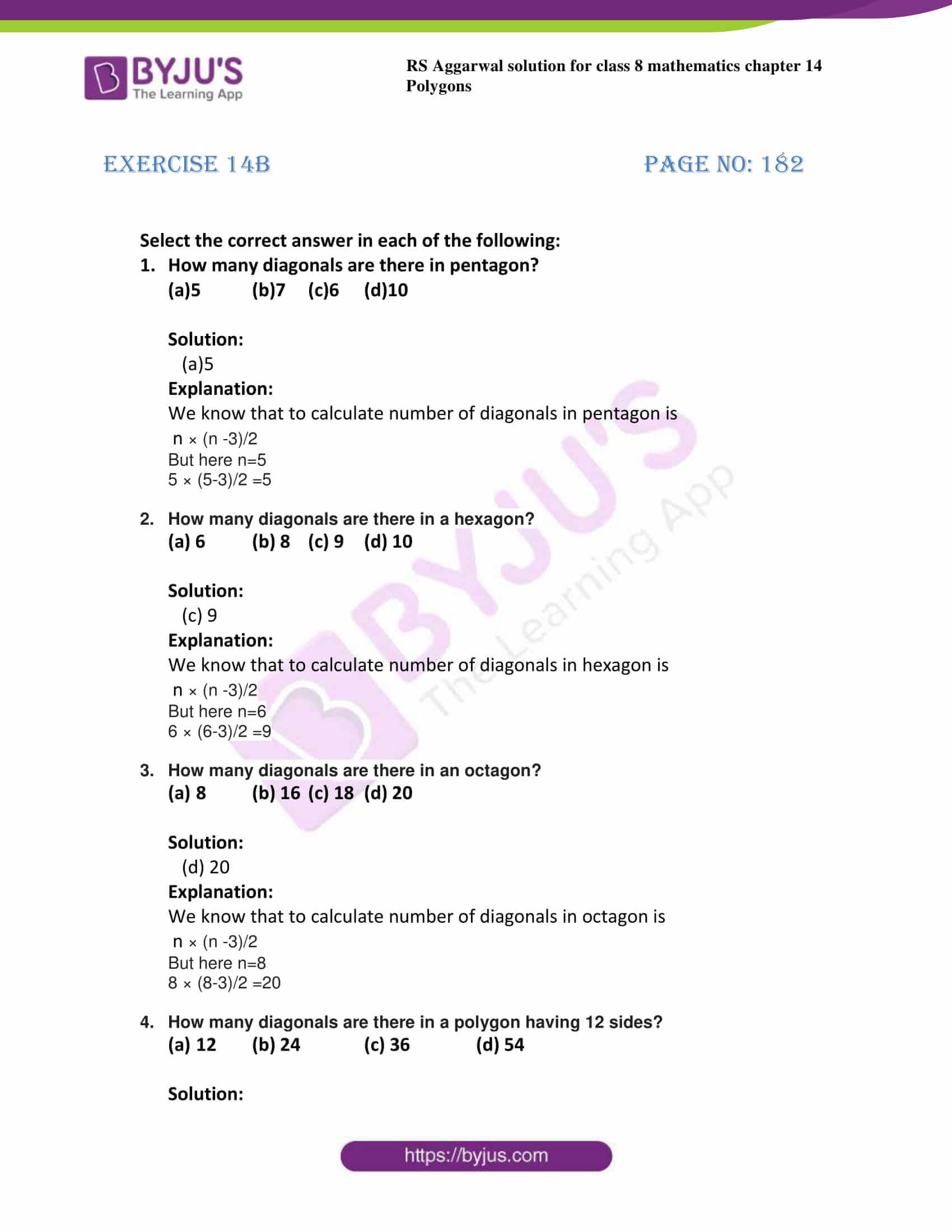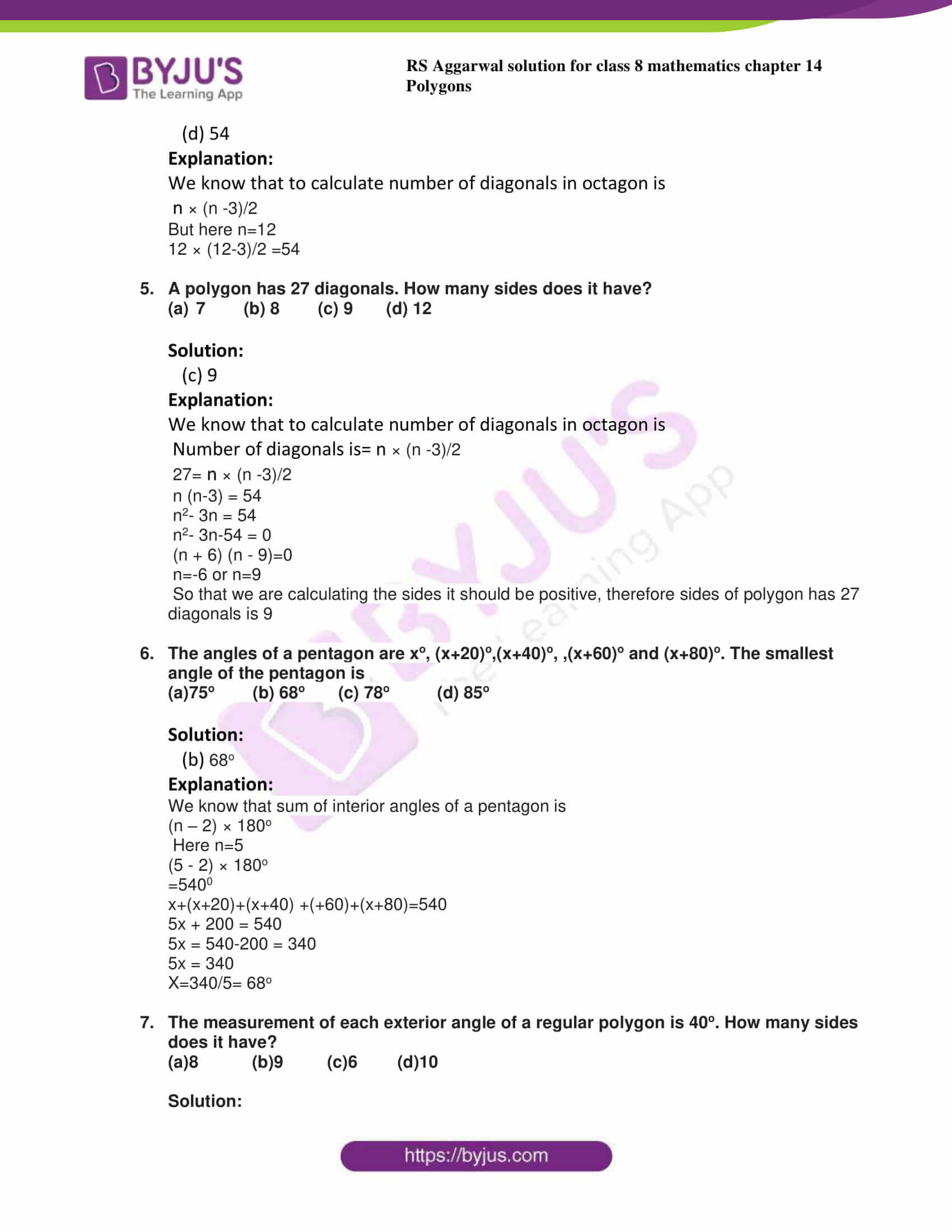# RS Aggarwal Solutions for Class 8 Maths Chapter 14 - Polygons Exercise 14B

Students can download the RS Aggarwal Solutions for the Exercise 14B of Class 8 Maths Chapter 14, Polygons from the available link given below. BYJU’S experts have solved the questions present in the Exercise 14B in Maths, and this will help students in solving this exercise without any dilemmas. This exercise holds objective questions which enhance the knowledge of the learner. By practising the RS Aggarwal Solutions for class 8, students will be able to grasp the concepts entirely. Hence, whose aim is to excel in the examinations are advised to go through RS Aggarwal Solutions for Class 8.

## Download PDF of RS Aggarwal Solutions for Class 8 Maths Chapter 14 – Polygons Exercise 14B### Access answers to Maths RS Aggarwal Solutions for Class 8 Chapter 14 – Polygons Exercise 14B

Select the correct answer in each of the following:

1. How many diagonals are there in pentagon?

(a)5 (b)7 (c)6 (d)10

Solution:

(a)5

Explanation:

We know that to calculate number of diagonals in pentagon is

n × (n -3)/2

But here n=5

5 × (5-3)/2 =5

2. How many diagonals are there in a hexagon?

(a) 6 (b) 8 (c) 9 (d) 10

Solution:

(c) 9

Explanation:

We know that to calculate number of diagonals in hexagon is

n × (n -3)/2

But here n=6

6 × (6-3)/2 =9

3. How many diagonals are there in an octagon?
a)8 (b) 16 (c) 18 (d) 20

Solution:

(d) 20

Explanation:

We know that to calculate number of diagonals in octagon is

n × (n -3)/2

But here n=8

8 × (8-3)/2 =20

4. How many diagonals are there in a polygon having 12 sides?
a)12 (b) 24 (c) 36 (d) 54

Solution:

(d) 54

Explanation:

We know that to calculate number of diagonals in octagon is

n × (n -3)/2

But here n=12

12 × (12-3)/2 =54

5. A polygon has 27 diagonals. How many sides does it have?
a)7 (b) 8 (c) 9 (d) 12

Solution:

(c) 9

Explanation:

We know that to calculate number of diagonals in octagon is

Number of diagonals is= n × (n -3)/2

27= n × (n -3)/2

n (n-3) = 54

n2– 3n = 54

n2– 3n-54 = 0

(n + 6) (n – 9)=0

n=-6 or n=9

So that we are calculating the sides it should be positive, therefore sides of polygon has 27 diagonals is 9

6. The angles of a pentagon are xo, (x+20)o,(x+40)o, ,(x+60)o and (x+80)o. The smallest angle of the pentagon is

(a)75o (b) 68o (c) 78o (d) 85o

Solution:

(b) 68o

Explanation:

We know that sum of interior angles of a pentagon is

(n – 2) × 180o

Here n=5

(5 – 2) × 180o

=5400

x+(x+20)+(x+40) +(+60)+(x+80)=540

5x + 200 = 540

5x = 540-200 = 340

5x = 340

X=340/5= 68o

7. The measurement of each exterior angle of a regular polygon is 40o. How many sides does it have?

(a)8 (b)9 (c)6 (d)10

Solution:

(b)9

Explanation:

Given exterior angle= 40o

But we know that Number of sides = 360/ exterior angle

Number of sides = 360/40=9

## RS Aggarwal Solutions for Class 8 Maths Chapter 14 – Polygons Exercise 14B

Exercise 14B of RS Aggarwal Solutions for Chapter 14, Polygons deals with the topics related to polygons and exterior, interior angles of polygons. Some of the topics focused prior to exercise 14B include the following.

• Formula to calculate the exterior angle of a polygon
• Formula to calculate the interior angle of a polygon
• Formula to calculate the sum of all exterior angles of a polygon
• Formula to calculate the sum of all interior angles of a polygon
• Formula to calculate the number of diagonals in a polygon

The RS Aggarwal Solutions can help the students in practising and learning each and every concept as it provides solutions to all questions asked in the RS Aggarwal textbook. Those who aim to score high in the Maths of class 8 are advised to practice all the questions present in RS Aggarwal as many times as possible.